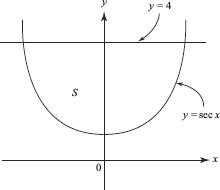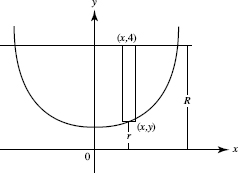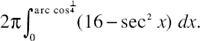# AP Calculus BC Question 165: Answer and Explanation

### Test Information

Question: 165

2.The region S in the figure above is bounded by y = sec x and y = 4. What is the volume of the solid formed when S is rotated about the x-axis?

• A. 108.177
• B. 39.867
• C. 53.126
• D. 54.088